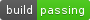Functions for plotting area-proportional two- and three-way Venn diagrams in matplotlib.

## Project descriptionRoutines for plotting area-weighted two- and three-circle venn diagrams.

## Installation

The simplest way to install the package is via easy_install or pip:

\$ easy_install matplotlib-venn

• numpy,

• scipy,

• matplotlib.

## Usage

The package provides four main functions: venn2, venn2_circles, venn3 and venn3_circles.

The functions venn2 and venn2_circles accept as their only required argument a 3-element list (Ab, aB, AB) of subset sizes, e.g.:

venn2(subsets = (3, 2, 1))

and draw a two-circle venn diagram with respective region areas. In the particular example, the region, corresponding to subset A and not B will be three times larger in area than the region, corresponding to subset A and B. Alternatively, you can simply provide a list of two set or Counter (i.e. multi-set) objects instead (new in version 0.7), e.g.:

venn2([set(['A', 'B', 'C', 'D']), set(['D', 'E', 'F'])])

Similarly, the functions venn3 and venn3_circles take a 7-element list of subset sizes (Abc, aBc, ABc, abC, AbC, aBC, ABC), and draw a three-circle area-weighted venn diagram. Alternatively, you can provide a list of three set or Counter objects (rather than counting sizes for all 7 subsets).

The functions venn2_circles and venn3_circles draw just the circles, whereas the functions venn2 and venn3 draw the diagrams as a collection of colored patches, annotated with text labels. In addition (version 0.7+), functions venn2_unweighted and venn3_unweighted draw the Venn diagrams without area-weighting.

Note that for a three-circle venn diagram it is not in general possible to achieve exact correspondence between the required set sizes and region areas, however in most cases the picture will still provide a decent indication.

The functions venn2_circles and venn3_circles return the list of matplotlib.patch.Circle objects that may be tuned further to your liking. The functions venn2 and venn3 return an object of class VennDiagram, which gives access to constituent patches, text elements, and (since version 0.7) the information about the centers and radii of the circles.

Basic Example:

from matplotlib_venn import venn2
venn2(subsets = (3, 2, 1))

For the three-circle case:

from matplotlib_venn import venn3
venn3(subsets = (1, 1, 1, 2, 1, 2, 2), set_labels = ('Set1', 'Set2', 'Set3'))

A more elaborate example:

from matplotlib import pyplot as plt
import numpy as np
from matplotlib_venn import venn3, venn3_circles
plt.figure(figsize=(4,4))
v = venn3(subsets=(1, 1, 1, 1, 1, 1, 1), set_labels = ('A', 'B', 'C'))
v.get_patch_by_id('100').set_alpha(1.0)
v.get_patch_by_id('100').set_color('white')
v.get_label_by_id('100').set_text('Unknown')
v.get_label_by_id('A').set_text('Set "A"')
c = venn3_circles(subsets=(1, 1, 1, 1, 1, 1, 1), linestyle='dashed')
c.set_lw(1.0)
c.set_ls('dotted')
plt.title("Sample Venn diagram")
plt.annotate('Unknown set', xy=v.get_label_by_id('100').get_position() - np.array([0, 0.05]), xytext=(-70,-70),
ha='center', textcoords='offset points', bbox=dict(boxstyle='round,pad=0.5', fc='gray', alpha=0.1),
plt.show()

An example with multiple subplots (new in version 0.6):

from matplotlib_venn import venn2, venn2_circles
figure, axes = plt.subplots(2, 2)
venn2(subsets={'10': 1, '01': 1, '11': 1}, set_labels = ('A', 'B'), ax=axes)
venn2_circles((1, 2, 3), ax=axes)
venn3(subsets=(1, 1, 1, 1, 1, 1, 1), set_labels = ('A', 'B', 'C'), ax=axes)
venn3_circles({'001': 10, '100': 20, '010': 21, '110': 13, '011': 14}, ax=axes)
plt.show()

Perhaps the most common use case is generating a Venn diagram given three sets of objects:

set1 = set(['A', 'B', 'C', 'D'])
set2 = set(['B', 'C', 'D', 'E'])
set3 = set(['C', 'D',' E', 'F', 'G'])

venn3([set1, set2, set3], ('Set1', 'Set2', 'Set3'))
plt.show()

## Questions

• If you ask your questions at StackOverflow and tag them matplotlib-venn, chances are high you’ll get an answer from the maintainer of this package.

## Project details

This version0.11.90.11.80.11.70.11.60.11.50.11.40.11.30.11.20.11.10.110.100.90.80.70.60.50.40.30.2

Uploaded source